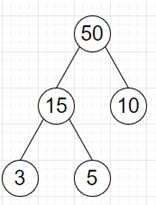# Program to find sum of minimum trees from the list of leaves in python

Suppose we have a list of numbers called nums. This list is representing the leaf nodes in inorder traversal of a tree. Here the internal nodes have have 2 children and their value is same as the product of the largest leaf value of its left subtree and the largest leaf value of its right subtree. We have to find the sum of the tree with the minimum sum of its values

So, if the input is like nums = [3, 5, 10], then the output will be 83.To solve this, we will follow these steps:

• res := sum of all elements in nums
• while size of nums > 1, do
• i := index of minimum element of nums
• left := nums[i - 1] when i > 0 otherwise infinity
• right := nums[i + 1] when i < size of nums - 1 otherwise infinity
• res := res + (minimum of left and right) * ith element of nums, then delete ith element from nums
• return res

Let us see the following implementation to get better understanding:

## Example Code

Live Demo

class Solution:
def solve(self, nums):
res = sum(nums)
while len(nums) > 1:
i = nums.index(min(nums))
left = nums[i - 1] if i > 0 else float("inf")
right = nums[i + 1] if i < len(nums) - 1 else float("inf")
res += min(left, right) * nums.pop(i)

return res

ob = Solution()
nums = [3, 5, 10]
print(ob.solve(nums))

## Input

[3, 5, 10]

## Output

83